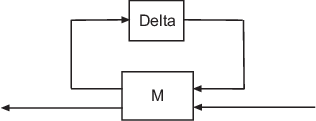Documentation

# lftdata

Decompose uncertain objects into fixed certain and normalized uncertain parts

## Syntax

```[M,Delta] = lftdata(A);
[M,Delta] = lftdata(A,List);
[M,Delta,Blkstruct] = lftdata(A);
[M,Delta,Blkstruct,Normunc] = lftdata(A);
```

## Description

`lftdata ` decomposes an uncertain object into a fixed certain part and a normalized uncertain part. `lftdata` can also partially decompose an uncertain object into an uncertain part and a normalized uncertain part. Uncertain objects (`umat, ufrd, uss`) are represented as certain (i.e., not-uncertain) objects in feedback with block-diagonal concatenations of uncertain elements.

`[M,Delta] = lftdata(A)` separates the uncertain object `A` into a certain object `M` and a normalized uncertain matrix `Delta` such that `A` is equal to `lft(Delta,M)`, as shown below.If `A` is a `umat`, then `M` will be `double`; if `A` is a `uss`, then `M` will be `ss`; if `A` is a `ufrd`, then `M` will be `frd`. In all cases, `Delta` is a `umat`.

`[M,Delta] = lftdata(A,List)` separates the uncertain object `A` into an uncertain object `M`, in feedback with a normalized uncertain matrix `Delta`. `List` is a cell (or char) array of names of uncertain elements of `A` that make up `Delta`. All other uncertainty in `A` remains in `M`.

`lftdata(A,fieldnames(A.Uncertainty))` is the same as `lftdata(A)`.

`[M,DELTA,BLKSTRUCT] = lftdata(A)` returns an N-by-1 structure array `BLKSTRUCT`, where `BLKSTRUCT(i)` describes the `i`-th normalized uncertain element. This uncertainty description can be passed directly to the low-level structured singular value analysis function `mussv`.

`[M,DELTA,BLKSTRUCT,NORMUNC] = lftdata(A)` returns the cell array `NORMUNC` of normalized uncertain elements. Each normalized element has `'Normalized'` appended to its original name to avoid confusion. Note that `lft(blkdiag(NORMUNC{:}),M)` is equivalent to `A`.

## Examples

Create an uncertain matrix `A` with 3 uncertain parameters `p1, p2` and `p3`. You can decompose `A` into its certain, `M`, and normalized uncertain parts, `Delta`.

```p1 = ureal('p1',-3,'perc',40); p2 = ucomplex('p2',2); A = [p1 p1+p2;1 p2]; [M,Delta] = lftdata(A); ```

You can inspect the difference between the original uncertain matrix, `A`, and the result formed by combining the two results from the decomposition.

```simplify(A-lft(Delta,M)) ans = 0 0 0 0 M M = 0 0 1.0954 1.0954 0 0 0 1.0000 1.0954 1.0000 -3.0000 -1.0000 0 1.0000 1.0000 2.0000 ```

You can check the worst-case norm of the uncertain part using `wcnorm`. Compare samples of the uncertain part `A` with the uncertain matrix `A`.

```wcn = wcnorm(Delta) wcn = lbound: 1.0000 ubound: 1.0001 usample(Delta,5) ans(:,:,1) = 0.8012 0 0 0.2499 + 0.6946i ans(:,:,2) = 0.4919 0 0 0.2863 + 0.6033i ans(:,:,3) = -0.1040 0 0 0.7322 - 0.3752i ans(:,:,4) = 0.8296 0 0 0.6831 + 0.1124i ans(:,:,5) = 0.6886 0 0 0.0838 + 0.3562i ```

### Uncertain Systems

Create an uncertain matrix `A` with 2 uncertain real parameters `v1` and `v2` and create an uncertain system `G` using `A` as the dynamic matrix and simple matrices for the input and output.

```A = [ureal('p1',-3,'perc',40) 1;1 ureal('p2',-2)]; sys = ss(A,[1;0],[0 1],0); sys.InputGroup.ActualIn = 1; sys.OutputGroup.ActualOut = 1; ```

You can decompose `G` into a certain system, `Msys`, and a normalized uncertain matrix, `Delta`. You can see from `Msys` that it is certain and that the input and output groups have been adjusted.

```[Msys,Delta] = lftdata(sys); Msys a = x1 x2 x1 -3 1 x2 1 -2 b = u1 u2 u3 x1 1.095 0 1 x2 0 1 0 c = x1 x2 y1 1.095 0 y2 0 1 y3 0 1 d = u1 u2 u3 y1 0 0 0 y2 0 0 0 y3 0 0 0 Input groups: Name Channels ActualIn 3 p1_NC 1 p2_NC 2 Output groups: Name Channels ActualOut 3 p1_NC 1 p2_NC 2 Continuous-time model. ```

You can compute the norm on samples of the difference between the original uncertain matrix and the result formed by combining `Msys` and `Delta`.

```norm(usample(sys-lft(Delta,Msys),'p1',4,'p2',3),'inf') ans = 0 0 0 0 0 0 0 0 0 0 0 0 ```

### Partial Decomposition

Create an uncertain matrix `A` and derive an uncertain matrix `B` using an implicit-to-explicit conversion, `imp2exp`. Note that `B` has 2 uncertain parameters `R` and `K`. You can decompose `B` into certain, `M`, and normalized uncertain parts, `Delta`.

```R = ureal('R',1,'Percentage',[-10 40]); K = ureal('K',2e-3,'Percentage',[-30 30]); A = [1 -R 0 -K;0 -K 1 0]; Yidx = [1 3]; Uidx = [4 2]; B = imp2exp(A,Yidx,Uidx); [M,Delta] = lftdata(B); ```

The same operation can be performed by defining the uncertain parameters, `K` and `R`, to be extracted.

```[MK,DeltaR] = lftdata(B,'R'); MK UMAT: 3 Rows, 3 Columns K: real, nominal = 0.002, variability = [-30 30]%, 2 occurrences [MR,DeltaK] = lftdata(B,'K'); MR UMAT: 4 Rows, 4 Columns R: real, nominal = 1, variability = [-10 40]%, 1 occurrence simplify(B-lft(Delta,M)) ans = 0 0 0 0 simplify(B-lft(DeltaR,MK)) ans = 0 0 0 0 simplify(B-lft(DeltaK,MR)) ans = 0 0 0 0 ```

Sample and inspect the uncertain part as well as the difference between the original uncertain matrix and the sampled matrix. You can see the result formed by combining the two results from the decomposition.

```[Mall,Deltaall] = lftdata(B,{'K';'R'}); simplify(Mall)-M ans = 0 0 0 0 0 0 0 0 0 0 0 0 0 0 0 0 0 0 0 0 0 0 0 0 0 ```